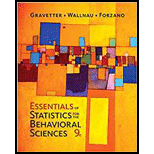Chapter 3, Problem 11P### Essentials of Statistics for The B...

9th Edition
Frederick J Gravetter + 2 others
ISBN: 9781337098120

#### Solutions

Chapter
Section### Essentials of Statistics for The B...

9th Edition
Frederick J Gravetter + 2 others
ISBN: 9781337098120
Textbook Problem

# A sample of n = 8 scores has a mean of M = 7. If one score is changed from X = 20 to X = 4, what is the value of the new sample mean?

To determine

To Find: The value of new sample mean when a score is changed from 20 to 4.

Explanation

Given info:

A sample of 8 scores has mean 7.

Calculations:

Consider the first 6 scores is x1x2x3x4x5x6 and the 7th score is, x7=20 .

The formula for sample mean is,

M=Xn

Substitute the values X=x1+x2+x3+x4+x5+x6+20 , M=7 and n=8 in the above formula

7=x1+x2+x3+x4+x5+x6+20856=x1+x2+x3+x4+x5+x6+2036=x1+x

### Still sussing out bartleby?

Check out a sample textbook solution.

See a sample solution

#### The Solution to Your Study Problems

Bartleby provides explanations to thousands of textbook problems written by our experts, many with advanced degrees!

Get Started

## Additional Math Solutions

#### Find more solutions based on key concepts